# DeBroglie Wavelength

A convenient form for the DeBroglie wavelength expression is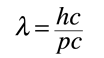where hc = 1239.84 eV nm and pc is expressed in electron volts.

This is particularly appropriate for comparison with photon wavelengths since for the photon, pc=E and a 1 eV photon is seen immediately to have a wavelength of 1240 nm. For massive particles with kinetic energy KE which is much less than their rest mass energies:...

### Show

For an electron with KE = 1 eV and rest mass energy 0.511 MeV, the associated DeBroglie wavelength is 1.23 nm, about a thousand times smaller than a 1 eV photon. (This is why the limiting resolution of an electron microscope is much higher than that of an optical microscope.)

The following calculation uses the full relativistic expressions for kinetic energy, etc.

 For rest mass m0 = me =mp=x10^kg
 where me= electron rest mass and mp= proton rest mass,
 and kinetic energy KE = eV =MeV = GeV = x10^joules

corresponding to velocity v =x10^m/s = c,

the corresponding DeBroglie wavelength is
λ = x10^m =nm =fermi.

Caution! Since this calculation was designed for speeds which are a significant fraction of the speed of light, it may lose some accuracy for very low speeds. Under those conditions, small differences between large numbers occur in the calculations. For low speed calculations like the baseball example, it might be more accurate to use the non-relativistic calculation.

### Low velocity calculation of DeBroglie wavelength

Index

 HyperPhysics***** Quantum Physics R Nave
Go Back

# Usefulness of the Quantity pc

The relativistic energy expression for a particle is: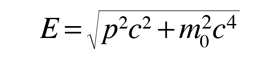which reduces to E = pc for photons . The quantity pc is proportional to the relativistic momentum but has energy units, and it is useful in many high energy calculations. Using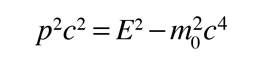andan expression for pc in terms of the relativistic kinetic energy can be obtained: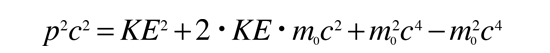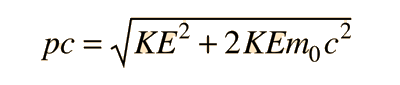Application to pion decay

For most ordinary processes the kinetic energy is much less than the rest mass energy, so the first term can usually be neglected. This leads to the useful approximation:For electron energies less than 20 keV and proton energies less than 38 MeV this expression has an error less than 1%.

 For extreme relativistic velocities where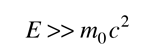then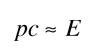For less than 1% error this requires electron energies over 3.2 MeV and proton energies over 5.8 GeV.

A useful application of the quantity pc is in the calculation of the velocity as a fraction of c.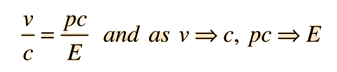with the limiting case applying for the momentum of photon.

 Calculation of DeBroglie wavelength
Index

 HyperPhysics***** Quantum Physics R Nave
Go Back